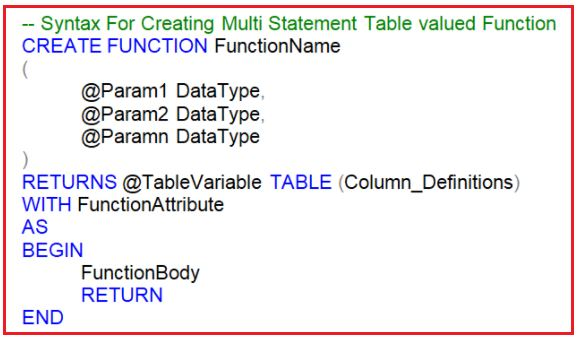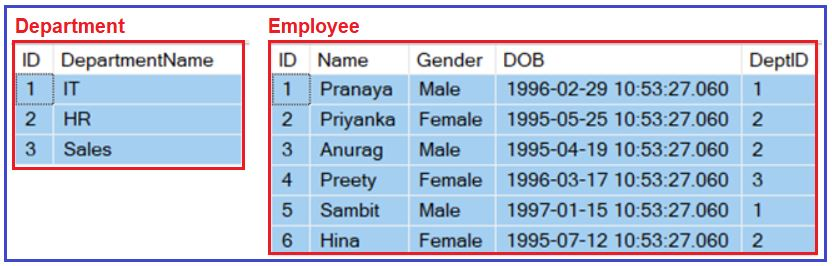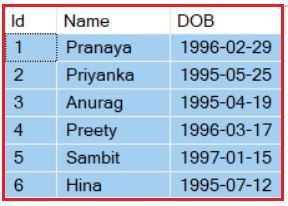# Multi Statement Table Valued Function in SQL Server

## Multi-Statement Table Valued Function in SQL Server

In this article, I am going to discuss the Multi-Statement Table Valued Function in SQL Server with Examples. Please read our previous article where we discussed Inline Table-Valued Function in SQL Server. There are two types of Table-Valued Functions in SQL Server i.e. Inline Table-Valued and Multi-Statement Table-Valued Function. In our previous article, we already discussed the Inline Table-Valued Function and in this article, we are going to discuss Multi-Statement Table-Valued Function in SQL Server.

##### Multi-Statement Table-Valued Function in SQL Server

The Multi-Statement Table Valued Function in SQL Server is the same as the Inline Table-Valued Function means it is also going to returns a table as an output but with the following differences.

1. The Multi-Statement Table-Valued Function body can contain more than one statement. In Inline Table-Valued Function, it contains only a single Select statement prepared by the return statement.
2. In Multi-Statement Table-Valued Function, the structure of the table returned from the function is defined by us. But, in Inline Table-Valued Function, the structure of the table is defined by the Select statement that is going to return from the function body.

The following image shows the syntax of the Multi-Statement Table-Valued Function in SQL Server.Note: In the case of Multi-Statement Table Valued Function in SQL Server, we need to define the structure of the table being return.

##### Example: Multi-Statement Table-Valued Function in SQL Server

Let us understand Multi-Statement Table-Valued Function comparing with the Inline Table-Valued Function in SQL Server with an example. We are going to use the following Department and Employee tables.Please use the below SQL Script to create and populate the Department and Employee tables with sample data.

```-- Create Department Table
CREATE TABLE Department
(
ID INT PRIMARY KEY,
DepartmentName VARCHAR(50)
)
GO

-- Populate the Department Table with test data
INSERT INTO Department VALUES(1, 'IT')
INSERT INTO Department VALUES(2, 'HR')
INSERT INTO Department VALUES(3, 'Sales')
GO

-- Create Employee Table
CREATE TABLE Employee
(
ID INT PRIMARY KEY,
Name VARCHAR(50),
Gender VARCHAR(50),
DOB DATETIME,
DeptID INT FOREIGN KEY REFERENCES Department(ID)
)
GO

-- Populate the Employee Table with test data
INSERT INTO Employee VALUES(1, 'Pranaya', 'Male','1996-02-29 10:53:27.060', 1)
INSERT INTO Employee VALUES(2, 'Priyanka', 'Female','1995-05-25 10:53:27.060', 2)
INSERT INTO Employee VALUES(3, 'Anurag', 'Male','1995-04-19 10:53:27.060', 2)
INSERT INTO Employee VALUES(4, 'Preety', 'Female','1996-03-17 10:53:27.060', 3)
INSERT INTO Employee VALUES(5, 'Sambit', 'Male','1997-01-15 10:53:27.060', 1)
INSERT INTO Employee VALUES(6, 'Hina', 'Female','1995-07-12 10:53:27.060', 2)
GO```
##### Example:

Let’s write both Inline and Multi-Statement Table-Valued functions in SQL Server that return the following output.###### Using Inline Table-Valued function
```-- Inline Table Valued function:
CREATE FUNCTION ILTVF_GetEmployees()
RETURNS TABLE
AS
RETURN (SELECT ID, Name, Cast(DOB AS Date) AS DOB
FROM Employee)```
Calling the Inline Table-Valued Function: SELECT * FROM ILTVF_GetEmployees()
###### Using Multi-Statement Table-Valued function
```-- Multi-statement Table Valued function:
CREATE FUNCTION MSTVF_GetEmployees()
RETURNS @Table Table (ID int, Name nvarchar(20), DOB Date)
AS
BEGIN
INSERT INTO @Table
SELECT ID, Name, Cast(DOB AS Date)
FROM Employee
Return
End```

Calling the Multi-statement Table Valued Function: SELECT * FROM MSTVF_GetEmployees()

##### What are the differences between Inline and Multi-Statement Table-Valued Functions in SQL Server?
1. In an Inline Table-Valued Function, the returns clause cannot define the structure of the table that the function is going to return whereas in the Multi-Statement Table-Valued Function the returns clause defines the structure of the table that the function is going to return.
2. The Inline Table-Valued Function cannot have BEGIN and END blocks whereas the Multi-Statement Table-Valued Function has the Begin and End blocks.
3. It is possible to update the underlying database table using the inline table-valued function but it is not possible to update the underlying database table using the multi-statement table-valued function.
4. Inline Table-Valued functions are better for performance than the Multi-Statement Table-Valued function. So, if the given task can be achieved using an Inline Table-Valued Function, then it is always preferred to use Inline Table-valued Function over the Multi-Statement Table-Valued function.

Reason For Better Performance: Internally SQL Server treats an Inline Table-Valued function much like a view and treats a Multi-Statement Table-Valued function as a stored procedure.

##### Example: Update underlying database table using the inline table-valued function in SQL Server

SELECT * FROM dbo.ILTVF_GetEmployees()
For the above function, Employee is the underlying database table.
UPDATE ILTVF_GetEmployees() SET Name=’Pranaya1′ WHERE ID= 1

The above update query will change the name Pranaya to Pranaya1, in the underlying table Employee. When we try to do the same thing with the multi-statement table-valued function, we will get an error stating ‘Object ‘MSTVF_GetEmployees’ cannot be modified.‘ The reason is that the multi-statement table-valued function did not get the data directly from the underlying database table instead it gets the data from the table variable.

Note: In Inline Table-Valued functions, we get the data directly from the underlying base table(s), and in the case of the Multi-Statement Table-Valued function, it gets the data from the table variable.

##### What is the Difference Between Functions and Procedures in SQL Server?
1. A function must return a value, it is mandatory whereas a procedure returning a value is optional.
2. The procedure can have parameters of both input and output whereas a function can have only input parameters.
3. In a procedure, we can perform Select. Update, Insert and Delete operations whereas function can only be used to perform select operations. It cannot be used to perform Insert, Update, and Delete operations that can change the state of the database.
4. A procedure provides the options to perform Transaction Management, Error Handling, etc whereas these operations are not possible in a function.
5. We call a procedure using EXECUTE/ EXEC command whereas a function is called by using SELECT command only.
6. From a procedure, we can call another procedure or a function whereas from a function we can call another function but not a procedure.
7. User-Defined Functions can be used in the SQL statements anywhere in the WHERE/HAVING/SELECT section where as Stored procedures cannot be.